### Analog Ammeters & Voltmeters

1. PMMC: Permanent Magnetic Moving Coil Instrument

2. MI: Moving Coil Instruments

3. Dynamometers type Instruments

4. Rectifier Instruments

5. Induction Type Instruments

Ammeter:

• The ammeter measures electric current. It may be calibrated in amperes, milli amperes, or microamperes. In order to measure current, the ammeter must be placed in series with the circuit to be tested (as shown in below Figure ).

• Figures : Ammeter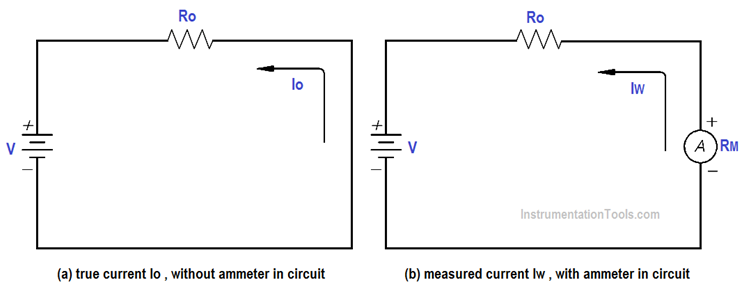• When an ammeter is placed in series with a circuit, it will increase the resistance of that circuit by an amount equal to the internal resistance of the meter Rm.The below Equation is the mathematical representation of the current without the meter installed.

Voltmeter:

• A simple DC voltmeter can be constructed by placing a resistor (RS), called a multiplier, in series with the ammeter meter movements,and marking the meter face to read voltage (as shown in Figure).Voltmeters are connected in parallel with the load (RL) being measured.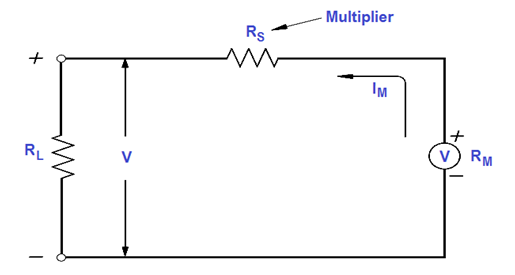• Figure: Simple Voltmeter

•  When constructing a voltmeter, the resistance of the multiplier must be determined to measure the desired voltage. The Equation is a mathematical representation of the voltmeter’s multiplier resistance.
V = ImRs + ImRm
ImRs = V – ImRm

• Where
V = voltage range desired
Im = meter current
Rm = meter resistance
Rs = multiplier resistance or series resistance

• The basic principle of working of ammeter and voltmeter is the same. In an ammeter the deflecting torque is produced by current which is to be measured. In voltmeter the deflecting torque is produced by current which is proportional to the voltage to be measured.

## Errors in Voltmeters and Ammeters

• Generally two types of errors are found common in voltmeter and ammeters which are due to the friction and temperature.

Friction:

• Friction is a force between two surfaces that are sliding, or trying to slide, across each other. For example, when you try to push a book along the floor, friction makes this difficult.

• Friction always works in the direction opposite to the direction in which the object is moving, or trying to move. To reduce the friction,the weight of the moving system must be measured as small as possible with the operating force. In the other words the ratio of torque to the weight must be very large.

Temperature:

• It is possible to reduce the error which is caused by the temperature change. The instrument must be mounted in such a position such that it is properly ventilated; a swamping resistance of low temperature coefficient can be connected in series with the coil to reduce the temperature effect on the temperature.
1. Permanent magnet moving coil instruments:
Several electrical machines and panels are fitted on board so that the ship can sail from one port to another, safely and efficiently. The electrical machinery and system require scheduled maintenance and checks to avoid any kind of breakdown during sailing. Different instruments are used on board for measuring several electrical parameters to analyze and keep these machinery in proper running condition. A permanent magnet moving coil (PMMC) is one such instrument which is popularly used onboard and has several applications.
• By using Permanent magnet moving coil instruments we can measure voltage or current.PMMC is used for the measurement of direct current or voltage.

• This instrument work on principle of D.C motor. According to this law when current carrying conductor lies in magnetic field then a force work current carrying conductor. This force is directly proportional to conductor current.

Construction:
The instrument has a moving coil of circular or rectangular wire, with the N turns suspended in the uniform, horizontal and radial magnetic field of a permanent magnet in the shape of a horse shoe. It is free to rotate about its vertical axis. The coil which is place around an iron core, which is spherical if the coil is circular, and if cylindrical if the coil is rectangular. since the coil is moving and the magnet is permanent, the instrument is called PMMC instrument.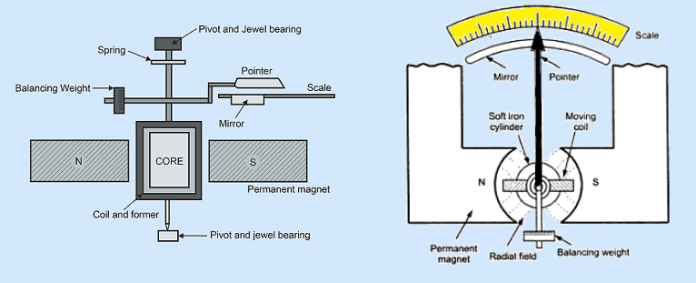Working:
When the current is passed through the coil it produces another magnetic field. So the interaction of this filed with the magnetic field of the Permanent Magnet produced an electromagnetic torque. The amount of force experienced by the coil is proportional to the current passing through the coil. This again becomes proportional to the measured quantity that is voltage or current. This electromagnetic torque is counter balanced by the mechanical torque of control springs attracted to the movable coil. The coil is wound on an aluminum former which moves in the magnetic field of the permanent magnet to provide eddy current damping. When the torque is balanced, the pointer attached to the moving coil will stop and its angular deflection will represent the amount of electrical current to be measured against a fixed reference or scale. The pointer deflection id proportional to the current passing through the coil.
The deflection torque is given by, Td = NBIA

Where,

N=Numbers of turns in the coil

B=Magnetic flux intensity in wb/m²

I=Current flowing through the coil

This torque will cause the coil to rotate until an equilibrium position is reached at an angle θ with its original orientation.

Electromagnetic torque = control spring torque
Td = Tc
NBIA = kcθ
θ = NBIA / kc
θ = KI
Thus the angular deflection is linearly proportional to the current (I) .

• High sensitivity

• Accurate and reliable

• Uniform scale

• Low power consumption

• Simple and effective damping mechanism

•  Somewhat costly as compared to moving-iron instruments.

• Cannot be used for A.C. measurements.

• Friction and temperature might introduce errors as in case of other instruments.

• Some errors are set in due to the aging of control springs and the permanent magnets.

1. ## Moving iron instruments

Construction and basic principle operation:
Moving-iron instruments are generally used to measure alternating voltages and currents. In moving-iron instruments the movable system consists of one or more pieces of specially-shaped soft iron, which are so pivoted as to be acted upon by the magnetic field produced by the current in the coil. There are two general types of moving-iron instruments namely:

1. Repulsion (or double iron)

2. Attraction (or single-iron) type

The brief description of different components of a moving-iron instrument is given below:

1. Moving element: A small piece of soft iron in the form of a vane or rod.

2. Coil: to produce the magnetic field due to current flowing through it and also to magnetize the iron pieces.

In repulsion type, a fixed vane or rod is also used and magnetized with the same polarity. Control torque is provided by spring or weight (gravity).

Damping torque is normally pneumatic, the damping device consisting of an air chamber and a moving vane attached to the instrument spindle.

Deflecting torque produces a movement on an aluminum pointer over a graduated scale.

The deflecting torque in any moving-iron instrument is due to forces on a small piece of magnetically ‘soft’ iron that is magnetized by a coil carrying the operating current. In repulsion type moving–iron instrument consists of two cylindrical soft iron vanes mounted within a fixed current-carrying coil.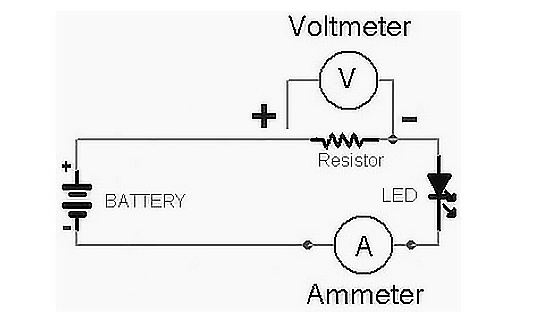Typical scheme of measuring electrical current and voltage

One iron vane is held fixed to the coil frame and the other is free to rotate, carrying with it the pointer shaft. Two irons lie in the magnetic field produced by the coil that consists of only a few turns if the instrument is an ammeter or of many turns if the instrument is a voltmeter.

Current in the coil induces both vanes to become magnetized and repulsion between the similarly magnetized vanes produces a proportional rotation. The deflecting torque is proportional to the square of the current in the coil, making the instrument reading a true ‘RMS’ quantity Rotation is opposed by a hairspring that produces the restoring torque. Only the fixed coil carries load current, and it is constructed so as to withstand high transient current.

Moving iron instruments have scales that are nonlinear and somewhat crowded in the lower range of calibration.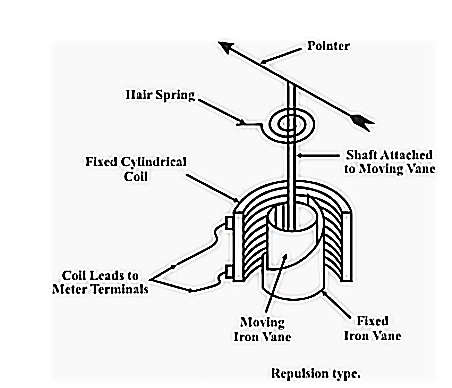Figure 1 – Repulsion moving iron-instrument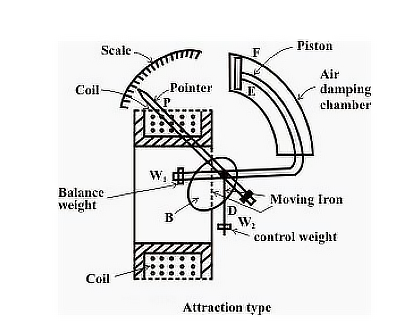Figure 2 – Attraction moving iron instrument

Measurement of Electric Voltage and Current

Moving iron instruments are used as Voltmeter and Ammeter only.

Both can work on AC as well as on DC.

Ammeter:

• Instrument used to measure current in the circuit.

• Always connected in series with the circuit and carries the current to be measured.

• This current flowing through the coil produces the desired deflecting torque.

• It should have low resistance as it is to be connected in series.

Voltmeter:

• Instrument used to measure voltage between two points in a circuit.

• Always connected in parallel.

• Current flowing through the operating coil of the meter produces deflecting torque.

• It should have high resistance. Thus a high resistance of order of kilo ohms is connected in series with the coil of the instrument.

• The instruments are suitable for use in AC and DC circuits.

• The instruments are robust, owing to the simple construction of the moving parts.

• The stationary parts of the instruments are also simple.

• Instrument is low cost compared to moving coil instruments.

• Torque/weight ratio is high, thus less frictional error.

Errors:

• Error due to variation in temperature

• Error due to friction is quite small as torque-weight ratio is high in moving coil instruments.

• Stray fields cause relatively low values of magnetizing force produced by the coil. Efficient magnetic screening is essential to reduce this effect.

• Error due to variation of frequency causes change of reactance of the coil and also changes the eddy currents induced in neighboring metal.

• Deflecting torque is not exactly proportional to the square of the current due to non-linear characteristics of iron material

• They are reliable.

• Simple in construction.

• Cheap in cost.

• Have high operating torque.

• Can be used for DC as well as AC measurement.

• The scales of these instruments are not uniform.

• Power construction is higher for low voltage range.

• Change in frequency also caused errors in AC measurement.

## Electrodynamometer type devices

An electrodynamometer type device is a transmission tool. The transfer tool is limited by d.c. source and used without any changes to a.c. ratings. Such a transfer tool has the same accuracy as a.c. and d.c. ratings. Electrodynamometer-type instruments are often used in accurate a.c. voltmeters and ammeters, not only at power line frequencies but also at low sound frequency frequencies. With some small adjustment, it can be used as a wattmeter for power measurements.

Why PMMC tools cannot be used for a.c. ratings?

• The PMMC tool cannot be claimed by a.c. currents or voltages. If a.c. the supply is provided on these tools, it will improve the torque alternating. Due to the short-term inertia of the moving system, the indicator will not follow the ever-changing torque and will fail to show any readings. So that the tool can read a.c. values, the magnetic field in the air space must change with the current change. This principle is applied to an electrodynamometer. Instead of a permanent magnet, an electrodynamometer-type tool is currently used under measurement to produce the required field flux. The diagram shows the construction of an electrodynamometer-type metal.

Construction: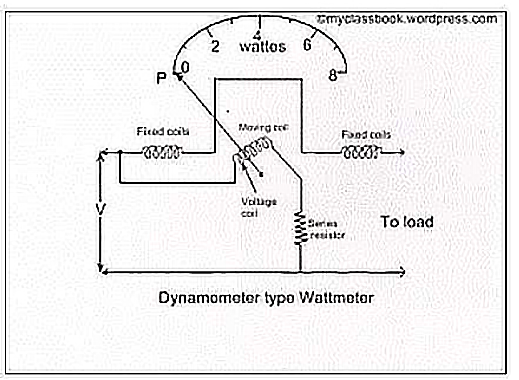• The different types of electrodynamometer types are:
1. Fixed Coils: The required field required for the operation of the metal is produced by fixed coils. The same field is located near the coil area due to the division of the coil into two sections. These coils are air cored. Flexible coils are damaged by fine wire for use as a voltmeter, while for ammeters and wattmeter's they are damaged by heavy wire. Coils usually have varnish. They are fastened in place to the base of the coil. This makes the construction stronger. Ceramic is often used for installed foundations. If metal parts were to be used they would weaken the constant coil field.

2. Moving coil: A moving coil is damaged as a reinforcing coil or other non-metallic material. If pre-metallic is used, it will add eddy currents to it. The construction of the moving coil is made simple and sturdy. Ine-air cored.

3. Control: Control torque is provided by springs. These springs act as a lead on a moving coil.

4. Moving System: A moving coil is mounted on an aluminum spindle. Contains opposition weights and a pointer. Sometimes suspension may be used, in the event of higher accuracy.

5. Damping: Slowing torque is provided by air collision, with pairs of aluminum vanes attached to the floor spindle. They walk in industrial rooms. Since the workspace can be distorted by eddy current damping, it is not rented.

6. Security: The field produced by these tools is very weak. Even the earth's magnetic field also protects us from harm. So protection is provided to protect it from magnetic fields. This is done by closing the casing high permeability alloy.

7. Cases and Scales: Standard laboratory tools are usually contained in polished wooden or metal cases. The case is supported by adjustable measuring screws. Air quality may be provided to ensure proper temperature. By using an electrodynamometer device as an ammeter, the constant and moving coils are connected to the series and carry the same current. The appropriate shunt is connected to these coils to limit the available power to the desired level. Electrodynamometer instruments can be used as a voltmeter by connecting fixed and moving coils in a series with high non-inductive resistance. The most accurate type of voltmeter. By using an electrodynamometer device as a wattmeter to measure power, fixed coils act as a current coil and must be connected to the series and load. Moving coils act as a voltage coil or pressure coil and should be connected to all terminals. The wattmeter indicates the power supply. When a current passes through a constant, moving coil, both coils produce magnetic fields. The field produced by a stable coil is equal to the current load while the field produced by the moving coil is equal to the electric field. Since the deviating torque is produced due to the interaction of these two fields, the deviation is accompanied by a given load force.

• As the tool has a Square Law response so it can be used in both dc and AC.

• These instruments have no current hysteresis and Eddy defects. ...

• An ammeter up to 10A and a voltmeter up to 600V can be built with precision distance accuracy.

• As air coil care coils, these steels have no hysteresis faults and current eddy faults.

• These instruments can be used in both a.c. and d.c. and as they have a degree of accuracy, they are very useful as transmission tools.

• The scale is not the same as the tool uses the Square Law response.

• These tools have a small amount of torque weight so the collision error is large.

• Due to the loss of motion of the moving system in these instruments is somehow higher than in other metals.

## Rectifier type Instruments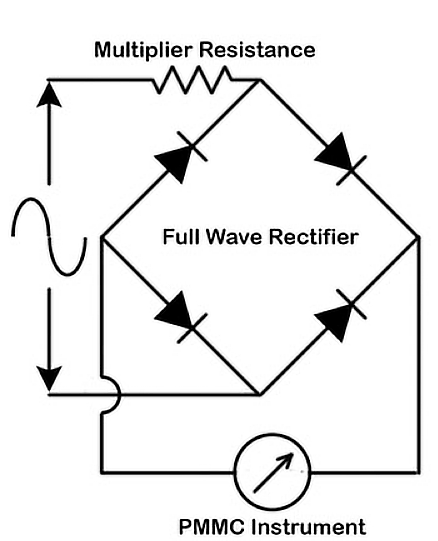It measures the alternating electric signal using the D.C. measurement tool. As the name implies, this Instrument  first adjusts the A.C signal to D.C. and then scales. Although it measures the adjusted A.C signal (D.C signal), but the metal scale is limited to A.C. The sensitivity of D ’Arsonval instrument is very high. But the D’Arsonval Instrument can only be measured in D.C. Thus, to use the A.C.’s D’Arsonval movement sensitivity, we use a Rectifier type Instrument.

The sensitivity of the repair kits is high compared to the moving coil and the Electro dynamo meter steel. Thus, it is used to measure current strength and voltage. Arrangement of the repair metal circuit shown in the figure below. The device uses four diodes that act as a correction element.